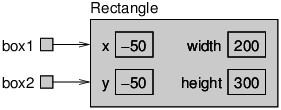# 1.6: Aliasing

$$\newcommand{\vecs}{\overset { \rightharpoonup} {\mathbf{#1}} }$$ $$\newcommand{\vecd}{\overset{-\!-\!\rightharpoonup}{\vphantom{a}\smash {#1}}}$$$$\newcommand{\id}{\mathrm{id}}$$ $$\newcommand{\Span}{\mathrm{span}}$$ $$\newcommand{\kernel}{\mathrm{null}\,}$$ $$\newcommand{\range}{\mathrm{range}\,}$$ $$\newcommand{\RealPart}{\mathrm{Re}}$$ $$\newcommand{\ImaginaryPart}{\mathrm{Im}}$$ $$\newcommand{\Argument}{\mathrm{Arg}}$$ $$\newcommand{\norm}{\| #1 \|}$$ $$\newcommand{\inner}{\langle #1, #2 \rangle}$$ $$\newcommand{\Span}{\mathrm{span}}$$ $$\newcommand{\id}{\mathrm{id}}$$ $$\newcommand{\Span}{\mathrm{span}}$$ $$\newcommand{\kernel}{\mathrm{null}\,}$$ $$\newcommand{\range}{\mathrm{range}\,}$$ $$\newcommand{\RealPart}{\mathrm{Re}}$$ $$\newcommand{\ImaginaryPart}{\mathrm{Im}}$$ $$\newcommand{\Argument}{\mathrm{Arg}}$$ $$\newcommand{\norm}{\| #1 \|}$$ $$\newcommand{\inner}{\langle #1, #2 \rangle}$$ $$\newcommand{\Span}{\mathrm{span}}$$$$\newcommand{\AA}{\unicode[.8,0]{x212B}}$$

Remember that when you assign an object to a variable, you are assigning a reference to an object. It is possible to have multiple variables that refer to the same object. The state diagram in Figure 10.6.1 shows the result.

Rectangle box1 = new Rectangle(0, 0, 100, 200);
Rectangle box2 = box1;Figure $$\PageIndex{1}$$: State diagram showing two variables that refer to the same object.

Notice how box1 and box2 are aliases for the same object, so any changes that affect one variable also affect the other. This example adds 50 to all four sides of the rectangle, so it moves the corner up and to the left by 50, and it increases the height and width by 100:

System.out.println(box2.width);
box1.grow(50, 50);
System.out.println(box2.width);


The first line displays 100, which is the width of the Rectangle referred to by box2. The second line invokes the grow method on box1, which stretches the Rectangle horizontally and vertically. The effect is shown in Figure 10.6.2.Figure $$\PageIndex{2}$$: State diagram showing the effect of invoking grow.

When we make a change using box1, we see the change using box2. Thus, the value displayed by the third line is 200, the width of the expanded rectangle.

This page titled 1.6: Aliasing is shared under a CC BY-NC-SA 3.0 license and was authored, remixed, and/or curated by Allen B. Downey (Green Tea Press) .